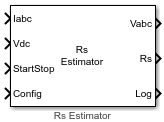# PMSM Rs Estimator

Estimate stator resistance (Rs) of permanent magnet synchronous motor (PMSM)

Since R2023a

•Libraries:
Motor Control Blockset / Parameter Estimation / PMSM Parameter Estimation

## Description

The PMSM Rs Estimator block computes the stator resistance of a PMSM using the phase currents, DC voltage across the motor, and the test configuration elements.

The block injects a voltage across the motor phases and measures the current response to estimate the motor resistance.

## Ports

### Input

expand all

The three-phase current response of the motor measured by the current sensors.

Note

Providing phase-c current input is optional. The block functionality is not impacted if you do not provide the phase-c current input.

Data Types: `single`

DC bus voltage that you provide to the inverter.

Data Types: `single`

Signal that either starts or stops Rs estimation:

• `1` (rising edge of a pulse) — This signal initiates the Rs estimation process.

• `0` (falling edge of a pulse) — This signal immediately stops the Rs estimation process. If you interrupt the estimation process abruptly before completion, the block does not compute or output the Rs value.

Data Types: `Boolean`

This table summarizes the expected configuration elements in the bus signal input.

CategoryConfiguration elementUnits

Elements for motor and inverter hardware

Number of pole pairs

-

Rated speed of motor

RPM

Rated current of motor

A

Maximum measurable inverter current

A

Board resistance

ohm

Current sense gain

Voltage sense gain

Parameter estimation sample time

seconds

Elements for estimating motor inductances (Ld and Lq)

Maximum frequency

Hz

Minimum frequency

Hz

Step for frequency sweep

Hz

Frequency step size for estimation

Hz

Number of frequencies for estimation

-

Elements for estimating back EMF constant (Ke), motor inertia (J), and friction constant (B)

Back EMF estimation time

seconds

Back EMF estimation speed

RPM

Specify current controller gains (off/on)

-

Proportional gain

-

Integral gain

-

Iq Reference current for FOC

PU current

Lower speed limit for inertia estimation

RPM

You can connect this input port to the `Config` output port of the PMSM Parameter Estimation Configurator block.

Data Types: `single`

### Output

expand all

Stator reference voltage components along the a, b, and c axes of the abc reference frame.

Data Types: `single`

Estimated stator resistance (in ohms) of the PMSM.

Data Types: `single`

Debug signals that the block provides for examination. The bus includes these debug signals.

Debug signalDescriptionUnits

Id

D-axis current

Amperes

Vd

D-axis voltage

Volts

status

`0`Did not complete Rs estimation

-

`1`Rs estimation completed successfully

-

Data Types: `single`

## Version History

Introduced in R2023a